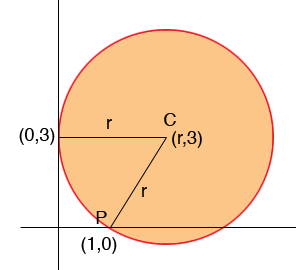SEARCH HOMEMath Central Quandaries & QueriesQuestion from Lindsay, a student: Hello. I'm trying to do a math problem and have searched the internet for equations but have come up empty handed. If you could help, that would be greatly appreciated! The problem is stated thus: A circle is tangent to the y-axis at y=3 and has one x-intercept at x=1. a. Determine the other x-intercept b. deduce the equation of the circle. Thanks!Hi Lindsay,

The first thing I would do is draw a diagram and label some points.Since the distance from the centre of the circle C to P is r

(r - 1)2 + (3 - 0)2 = r2

Solve for r.

I hope this helps,
PennyMath Central is supported by the University of Regina and The Pacific Institute for the Mathematical Sciences.